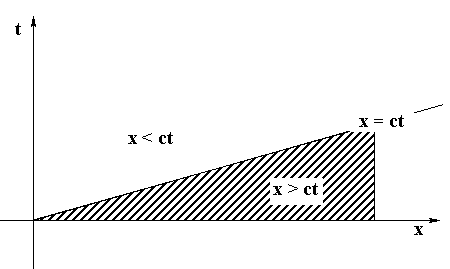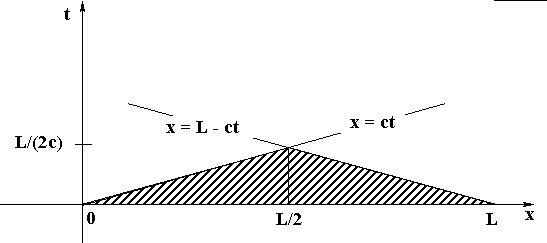# d'Alembert Solution of the Wave Equation

### Dr. R. L. Herman

This is a summary of solutions of the wave equation based upon the d'Alembert solution. This is meant to be a review of material already covered in class. We begin with the general solution and then specify initial and boundary conditions in later sections. This will show the tie between the various solutions covered in class, and some of those not covered in detail. We end with examples of animations done in Maple 6.

## 1  General Solution

 utt = c2uxx,
(1)
which was derived in class for small amplitude vibrations of a uniform string under a constant tension.

Making the substitutions x = x+ct and h = x-ct, this equation is transformed to

 uxh = 0.
(2)
This equation can be integrated to find solutions take the form of a sum of a wave traveling to the right and one traveling to the left:

 u(x,t) = F(x)+G(h),        or        u(x,t) = F(x+ct)+G(x-ct),
(3)
where F and G are arbitrary functions that can be determined from prescribed initial and boundary conditions. We will review these in the next sections.

## 2  Initial Conditions

The wave equation (1) is second order in time. Therefore, we need two initial conditions, specifying the initial position u(x,0) and the initial velocity ut(x,0) of each point on the string. Thus, we assume

 u(x,0) = f(x),        ut(x,0) = g(x).
(4)

Imposing these conditions on the general solution (3), we have

 F(x)+G(x) = f(x),
(5)
 c[F˘(x)-G˘(x) ] = g(x).
(6)

Integrating the second equation, we obtain a second equation for the unknown functions, F(x) and G(x).

 F(x)-G(x) = 1 c óő x 0 g(s) ds +F(0)-G(0).
(7)
Adding and subtracting to Equation (5), we find general forms for our unknown functions:

 F(x) = 1 2 f(x) + 1 2c óő x 0 g(s) ds + 1 2 [ F(0)-G(0) ],
(8)
 G(x) = 1 2 f(x) - 1 2c óő x 0 g(s) ds - 1 2 [ F(0)-G(0) ].
(9)

Now, the solution to the wave equation satisfying our initial conditions, can be written

 u(x,t)
 =
 F(x+ct)+G(x-ct)
 =
 1 2 [f(x+ct)+f(x-ct)] + 1 2c óő x+ct x-ct g(s) ds.
(10)
Note that F(0) and G(0) do not appear in this physical solution. Therefore, we will drop them in the rest of this discussion.

## 3  Boundary Conditions

The problem is that the solution in (10) only makes sense for the part of the interval [x-ct, x+ct] contained in the domain of f and g. This is fine if these domains are the entire real axis. However, there are problems for smaller domains. In such cases we will needed to make use of the boundary conditions to get to the solution of the initial-boundary value problem.

### 3.1  Infinite String

For f(x) and g(x) defined on -Ą < x < Ą, such as in the case of an infinite string, the solution (10) is well-defined.

 u(x,t)
 =
 F(x+ct)+G(x-ct)
 =
 1 2 [f(x+ct)+f(x-ct)] + 1 2c óő x+ct x-ct g(s) ds.
(11)

### 3.2  Semi-infinite String

For f(x) and g(x) defined on 0 Ł x < Ą, such as in the case of the semi-infinite string, the solution (10) is not well-defined. For positive c and t > 0, we have that f(x-ct) is not defined for x-ct < 0, or t > x/c. This also affects the range of integration over values where g is not defined.

This can be seen using characteristics in the xt-plane. Information from the point x = 0 can only propagate to the right at speed c. Drawing the line x = ct, we find that values for u(x,t) can only be obtained from the initial condition alone if the point (x,t) lies below the characteristic. In order to get values for points above the characteristics, we need to specify information, which will propagate from that region. Specifying values along the t-axis will provide such information. Namely, we need to specify a boundary condition at x = 0.

Two types of boundary conditions are that a) the end is fixed, u(0,t) = 0,  t ł 0, or b) the end is free ux(0,t) = 0,  t ł 0. We will treat each case separately.#### 3.2.1  Fixed End

For a fixed end, u(0,t) = 0,  t ł 0, we have

 0 = u(0,t) = F(ct)+G(-ct).
(12)
Letting z = -ct, this equation can be rewritten as
 G(z) = -F(-z),  z Ł 0.
(13)

This equation extends the range of G to negative values and can then be used to do the same for F. Inserting the expressions for F and G from Equations (8)-(9), we have

 G(z)
 =
 1 2 f(z) - 1 2c óő z 0 g(s) ds,
(14)
 -F(-z)
 =
 - 1 2 f(-z) - 1 2c óő -z 0 g(s) ds
 =
 - 1 2 f(-z) + 1 2c óő z 0 g(-s) ds
(15)

Comparing the expressions in (14) and (15), we see that we can satisfy (13) if

 f(z) = - f(-z),        and        g(z) = -g(-z).
(16)
In other words, we can extend the functions f and g to be odd functions with respect to x = 0.

#### 3.2.2  Free End

For a free end, ux(0,t) = 0,  t ł 0, we have

 0 = ux(0,t) = F˘(ct)+ G˘(-ct).
(17)
Letting z = -ct, this equation can be rewritten as
 G˘(z) = -F˘(-z),  z Ł 0.
(18)

Inserting the expressions for F and G from Equations (8)-(9), we have

 G˘(z)
 =
 1 2 f˘(z) - 1 2c g(z),
(19)
 -F˘(-z)
 =
 - 1 2 f˘(-z) - 1 2c g(-z).
(20)

Comparing these expressions, we see that we can satisfy (18) if

 f˘(z) = - f˘(-z),        and        g(z) = g(-z).
(21)
This means that the function f˘ needs to be an odd function with respect to x = 0. Thus, the functions f and g need to be even functions with respect to x = 0.

### 3.3  Finite String

In this case we have a physical string, which has a finite length l. We first consider the propagation of information from the initial conditions along the characteristics. f and g are defined for 0 Ł x Ł l. The general solution (10) is of the form F(x+ct)+G(x-ct). Thus, we can only compute F for 0 Ł x+ct Ł l and G for 0 Ł x-ct Ł l. For t ł 0, we then have t < [(l-x)/ c] and t < x/c, respectively.

The region defined by these inequalities is a triangular region. This region contains all points whose solution can be obtained using only the initial conditions. In order to obtain more information about the future values of the solution, we need to specify boundary conditions at x = 0 (to give G) and x = l (to give F).In either case we can specify fixed or free ends. We have seen previously what this means for x = 0:

 G(z)
 =
 -F(-z),  z Ł 0,     fixed end
(22)
 G˘(z)
 =
 -F˘(-z)  z Ł 0,     free end.
(23)

We now consider the conditions at x = l.

### 3.4  Conditions at x = l

#### 3.4.1  Fixed End

For a fixed end, u(l,t) = 0,  t ł 0, we have

 0 = u(l,t) = F(l+ct)+G(l-ct).
(24)
Letting z = l+ct, this equation can be rewritten as
 F(z) = -G(2l-z),  z ł l.
(25)

This equation extends the range of F to values l Ł z Ł 2l. Inserting the expressions for F and G from Equations (8)-(9), we have

 F(z)
 =
 1 2 f(z) + 1 2c óő z 0 g(s) ds,
(26)
 -G(2l-z)
 =
 - 1 2 f(2l-z) + 1 2c óő 2l-z 0 g(s) ds
 =
 - 1 2 f(2l-z) - 1 2c óő z 2l g(2l-t) dt.
(27)

Comparing these expressions, we see that we can satisfy (25) if

 f(2l-z) = - f(z),
(28)
and
 óő z 0 g(s) ds = - óő z 2l g(2l-t) dt.
(29)
Differentiating the last expression with respect to z, we obtain
 g(2l-z) = - g(z).
(30)
These conditions on f and g mean that we can extend these functions to l Ł z Ł 2l by performing and odd extension about x = l. This is very similar to the case encountered for a fixed end at x = 0.

#### 3.4.2  Free End

For a free end, ux(l,t) = 0,  t ł 0, we have

 0 = ux(l,t) = F˘(l+ct)+ G˘(l-ct).
(31)
Letting z = l+ct, this equation can be rewritten as
 F˘(z) = -G˘(2l-z),  z ł l.
(32)

Inserting the expressions for F and G from Equations (8)-(9), we have

 F˘(z)
 =
 1 2 f˘(z) + 1 2c g(z),
(33)
 -G˘(2l-z)
 =
 - 1 2 f˘(2l-z) + 1 2c g(2l-z).
(34)

Comparing these expressions, we see that we can satisfy (18) if

 f˘(z) = - f˘(2l-z),        and        g(z) = g(2l-z).
(35)
This means that the function f˘ needs to be an odd function with respect to x = l. Thus, the functions f and g need to be even functions with respect to x = l.

#### 3.4.3  Tying Loose Ends

Now all we need to do is look at the different possibilities for the ends. We could have two fixed ends, two free ends, or one free and one fixed. With each case we use the results for the type of extensions for f(x) and g(x). These will lead to certain types of periodic extensions for these functions, which can be used to rewrite the general solution as a well defined solution to the problem over the finite interval.

1. Two Fixed Ends

In this case we have the conditions

 f(x) = -f(-x),     -l Ł x Ł 0
 f(x) = -f(2l-x),     l Ł x Ł 2l.
(36)

Then

 f(x+2l) = -f(2l-(x+2l)) = - f(-x) = f(x).
Thus, we see that f and g need to be periodic odd extensions of the original functions with period 2l.

2. Two Free Ends

In this case we have the conditions

 f(x) = f(-x),     -l Ł x Ł 0
 f(x) = f(2l-x),     l Ł x Ł 2l.
(37)

Then

 f(x+2l) = f(2l-(x+2l)) = f(-x) = f(x).
Thus, we see that f and g need to be periodic even extensions of the original functions with period 2l.

3. One Fixed End and One Free End

In this case we either have the conditions (fixed at x = 0)

 f(x) = -f(-x),     -l Ł x Ł 0
 f(x) = f(2l-x),     l Ł x Ł 2l.
(38)
or we have the conditions (free at x = 0)

 f(x) = f(-x),     -l Ł x Ł 0
 f(x) = -f(2l-x),     l Ł x Ł 2l.
(39)

In the first case,

 f(x+4l)
 =
 f(2l-(x+4l)) = f(-x-2l)
 =
 -f(x+2l) = -f(2l-(x+l))
 =
 -f(-x) = f(x).
(40)
Thus, we see that f and g need to be periodic odd extensions of the original functions with period 4l, since f is an odd extension about x = 0.

Similarly, for the second case,

 f(x+4l)
 =
 -f(2l-(x+4l)) = -f(-x-2l)
 =
 -f(x+2l) = f(2l-(x+l))
 =
 f(-x) = f(x).
(41)
Thus, we see that f and g need to be periodic even extensions of the original functions with period 4l, since f is an even extension about x = 0.

### 3.5  Examples

Here are some examples in Maple:

Example 1 HTML version, Maple version (Examples for two fixed ends)

Example 2 HTML version, Maple version (Examples for one end fixed and the other free)

Example 3 HTML version, Maple version (Presentation of the odd extensions needed to solve the problem of two fixed ends with g(x)=0.)

File translated from TEX by TTH, version 2.25.
On 13 Sep 2000, 08:11.